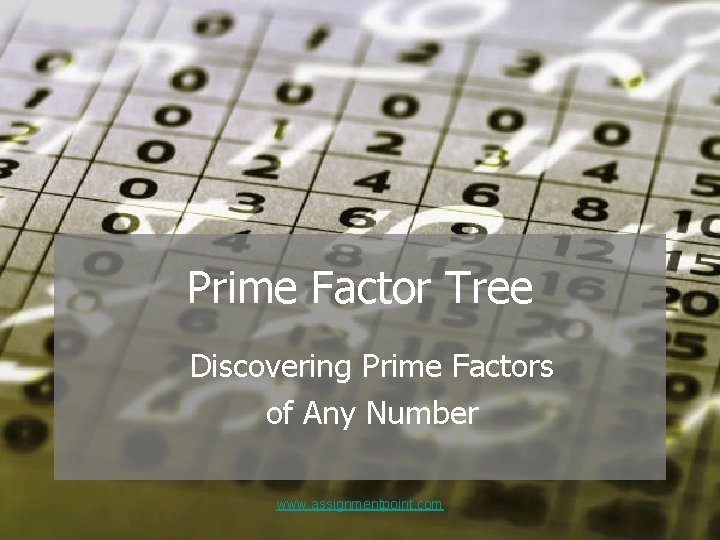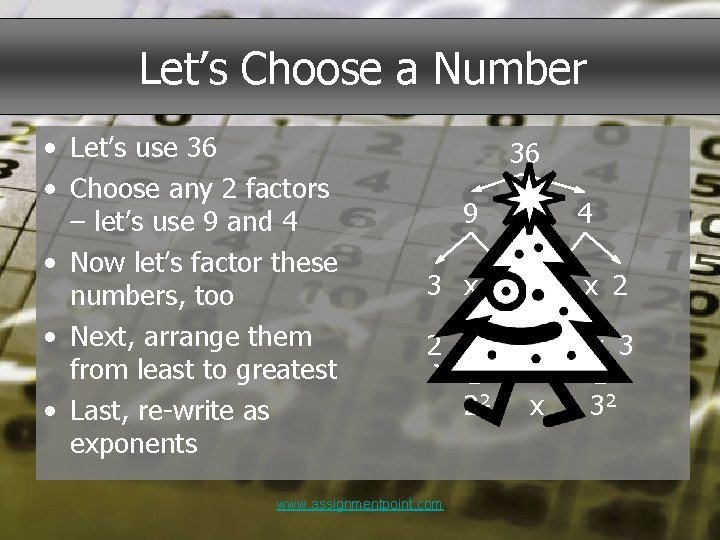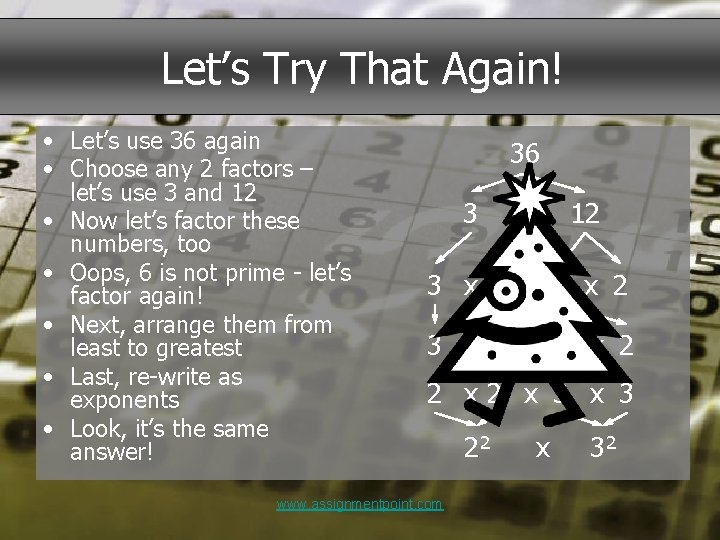# Prime Factor Tree Discovering Prime Factors of Any

• Slides: 5Prime Factor Tree Discovering Prime Factors of Any Number www. assignmentpoint. comDefinitions • Prime Number – An integer whose only factors are 1 and itself. • Factor – a number that can divide another number without a remainder. • Prime Factors – an expression of numbers that divides another integer without a remainder where all the factors are prime. www. assignmentpoint. comLet’s Choose a Number • Let’s use 36 • Choose any 2 factors – let’s use 9 and 4 • Now let’s factor these numbers, too • Next, arrange them from least to greatest • Last, re-write as exponents 36 9 x 4 3 x 3 2 x 2 3 x 3 www. assignmentpoint. com 22 x 32Let’s Try That Again! • Let’s use 36 again • Choose any 2 factors – let’s use 3 and 12 • Now let’s factor these numbers, too • Oops, 6 is not prime - let’s factor again! • Next, arrange them from least to greatest • Last, re-write as exponents • Look, it’s the same answer! 36 3 x 12 6 x 2 3 x 2 x 3 x 2 2 x 3 x 3 www. assignmentpoint. com 22 x 32Let’s Re-Cap • Composite numbers are made up of factors • In a Prime Factor Tree, the goal is to keep reducing each factor to its lowest possible prime factors • When you have all the prime factors identified, re-arrange them from least to greatest and re-write them as exponents www. assignmentpoint. com